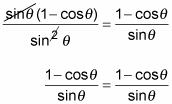##### Trigonometry Workbook For DummiesConjugates offer a great way to find trigonometry identities. In mathematics, a conjugate consists of the same two terms as the first expression, separated by the opposite sign. For instance, the conjugate of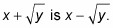In trig, multiplying the numerator and denominator of a fraction by a conjugate can create some really nice results.

For example, multiplying by a conjugate is a quick, easy way of solving the identity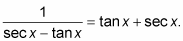1. Multiply the numerator and denominator of the fraction on the left by the conjugate of the denominator.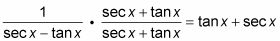2. The two denominators multiplied together are the difference of two squares.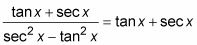3. Replace sec2 x in the denominator with its equivalent by using the Pythagorean identity.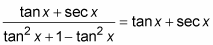4. Simplify the denominator by canceling out the two opposites.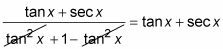In the next example, you have to decide which fraction to multiply the conjugate by. This example chooses the fraction on the right, because the conjugate of the numerator on the right is visible in the denominator on the left. Solve the identity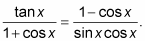1. Multiply the numerator and denominator of the fraction on the right by the conjugate of the numerator.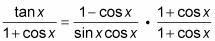2. Multiply the fractions together, keeping the parentheses in the denominator.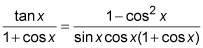3. Substitute the equivalent from the Pythagorean identity in the numerator of the fraction on the right. Then reduce the fraction.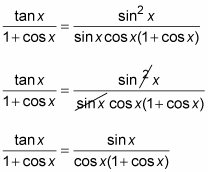4. Rewrite the fraction on the right as a product of two fractions, carefully arranging the factors.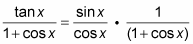5. Replace the first fraction on the right with its ratio-identity equivalent. Rewrite the expression as one fraction.The half-angle identity for the tangent function has two different forms. Multiplying by the conjugate is a good method for showing that these two forms are equivalent. The following example proves that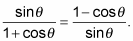1. Multiply the numerator and denominator of the fraction on the left by the conjugate of the denominator.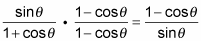2. Multiply the two denominators together, but leave the numerator in factored form.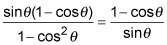3. Replace the denominator on the left with its equivalent by using the Pythagorean identity.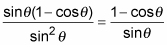4. Reduce the fraction on the left.